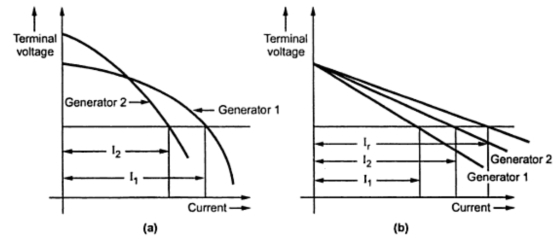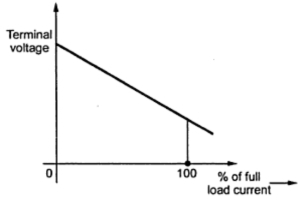### D.C. Shunt Generators in Parallel

For stable parallel operation, the most suitable type of d.c. generator is shunt generator as it has slightly drooping characteristics. If there is any tendency for a generator to supply more or less than its proper share of load it changes system voltage which certainly opposes this tendency. This restores the original division of load. Thus the shunt generators automatically remains in parallel, once they are paralleled.
If any of the generators is taken out of service then firstly its field is weakened while the field of other generators is strengthened till the ammeter associated with that generator reads zero. After this, the breaker and the concerned switch are opened to take the generator out of service. This procedure of connecting and disconnecting a generator from service prevents any shock and sudden disturbance to the prime mover or the system itself.
If field of any generator is weakened beyond certain extent then power will be delivered to it. It will then run as a motor in its original direction. The prime mover associated with it will be thus as a motor in its original direction. The prime mover associated with it will be thus driven due to the motoring action of the generator.
Consider voltage-current characteristics of a shunt generator as shown in the Fig. 1(a) and Fig1(b).Fig. 1

Two shunt generators are considered. For common terminal voltage, the two generators are supplying a current of I1 and I2 respectively. It can be seen that generator 2 has more dropping characteristics and supplied less current.
The load will be divided properly within the two generators at all the points provided their voltage characteristics are similar with each generator having same voltage drop from no load to full load.
If the two generators are having different kW ratings then they will share a load in proportion to their ratings with their external characteristics plotted with reference to percentage of full load current as shown in the Fig. 2.Fig. 2

If we consider two generators with their ratings as say 5o kW and 100 kW running in parallel and supplying a total load of 120 kW then generator 1 will supply a total load of 40 kW while the other one will supply 80 kW.
The combined characteristics of the generators can be obtained from the individual characteristics of the generators by adding the currents provided by each generator separately at equal voltage points. From this resultant characteristics the voltage or the currents provided by the generators can be obtained. If the generators are having straight line characteristics then these results can be obtained by doing the simple calculations instead of plotting graphs.
If the two generators having unequal no-load voltages are sharing a load then its analysis is as given below.

R1 = Armature resistance of generator 1
R2  = Armature resistance of generator2
V = Common terminal voltage

The bus bar voltage can be kept constant and the load can be transferred from generator 1 to 2 by increasing either Φ2 or N2 or by reducing N1 and Φ1 change in N1 and N2 can be achieved by changing the speeds of prime movers while by changing the shunt field rheostats the fluxes Φ1 and Φ2 can be varied.
In summary it should be kept in mind two shunt generators in parallel having no load voltages equal will share the load in such a ratio that the load current of each machine produces the same drop in each generator. If the generators are having unequal no-load voltages and operated in parallel then the load currents should produce sufficient voltage drops in each generator so as to keep the terminal voltage same. The generator having lower voltage drop takes more share of change in load. If the generators are working in parallel and having different power ratings but same voltage regulation will divide the load in proportion to their power ratings.